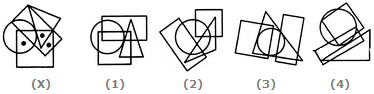# Non Verbal Reasoning - Dot Situation - Discussion

### Discussion :: Dot Situation - Section 1 (Q.No.4)

From amongst the figures marked (1), (2), (3) and (4), select the figure which satisfies the same conditions of placement of the dots as in figure (X).

4.

Select the figure which satisfies the same conditions of placement of the dots as in Figure-X.[A]. 1 [B]. 2 [C]. 3 [D]. 4

Explanation:

In fig. (X), one of the dots lies in the region common to the circle and the square only, another dot lies in the region common to the square, the triangle and the rectangle only and the third dot lies in the region common to the square and the rectangle only. In fig. (2) there is no region common to the square and the rectangle only, in fig. (3) there is no region common to the circle and the square only and in fig. (4) there is no region common to the square, the triangle and the rectangle only. Only fig. (1) consists of all the three types of regions.

 Sahil said: (Aug 22, 2013) Is the small white dot visible in the 1 between the square and rec is taken as the third dot?

 Sakshi said: (Jul 13, 2017) Where is the common dot between rectangle and square?

 Balamurali said: (Sep 23, 2019) There is a dot common to square and triangle where will you place it? Please explain it.